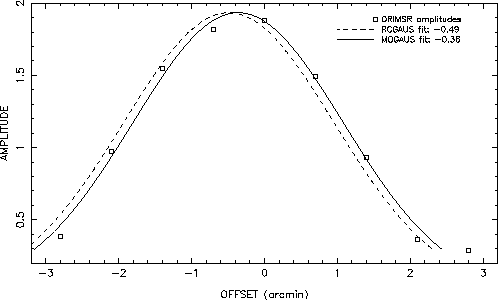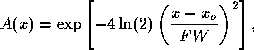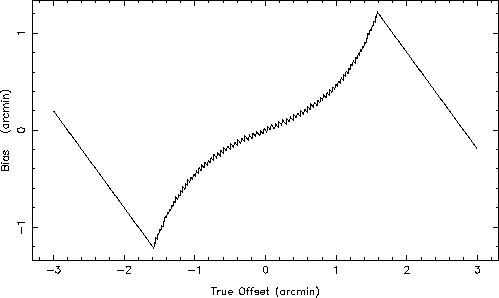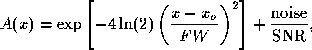# Systematic Errors in Pointing Determination Using the CROSS Program

Arie W. Grossman
University of Maryland
April 16, 1991

## Abstract

It is shown that pointing offsets determined by program CROSS exhibit systematic errors as large as 70%. These errors are due to improperly fitting a Gaussian to the offset measurements in subroutine RCGAUS. The results of fitting model Gaussians with and without additive noise show that a non-linear fitting method based on the Levenberg-Marquardt is superior to the the cross-correlation method of RCGAUS.

## Introduction

Pointing constants of the BIMA interferometer are usually derived from a combination of optical and radio pointing. As has been pointed out in the past, (see BIMA memo #2 "Hat Creek Interferometer Pointing Fitting", M. Wright, 3-Jan-1990) parameters derived from optical pointing are reproducible, however, there is a large dispersion in the radio determined pointing. We investigate the radio pointing methodology in an effort to understand this discrepancy.

Radio pointing is usually measured with the program CROSS. This program does a series of interferometer observations of a source, while offsetting the pointing of one antenna in a cross pattern. That is, the antenna is first offset in azimuth while the elevation is held fixed, then the antenna is offset in elevation while the azimuth is held fixed. The other antenna is held fixed on the source. Thus each leg of the cross provides an independent estimate of the offset in either elevation or azimuth. The number of steps in the cross pattern and the step size are controlled by user inputs. Each leg of the cross is independently fit to a 1-dimensional gaussian using a modified cross-correlation algorithm in subroutine RCGAUS.

CROSS is usually executed inside the DCL command file POINT.COM or APOINT.COM which alternates among bright continuum and SIO maser sources. Each object is observed for three trials of CROSS, alternately holding one of three antennas fixed. The amplitudes are usually measured for 10 to 20 seconds at 9 positions each offset by 0.7 arcminutes (e.g. at offsets of -2.8, -2.1, -1.4, -0.7, 0.0, 0.7, 1.4, 2.1, 2.8 arcmin). The complete cycle of three CROSS measurements usually takes about 18 to 30 minutes per source.

## Example Pointing Fitting

Consider an elevation pointing measurement done on March 14, 1991 on source ORIMSR. The amplitude was measured at 9 positions which are summarized in Table 1 and fig. 1. These measurements are analyzed by fitting a Gaussian using three different techniques. The three methods are described in detail in the appendices. Here it is sufficient to know that RCGAUS is the subroutine used by program CROSS. In addition we use a cross-correlation fitting program (CCGAUS) which provides a quick and dirty fit to position only, as well as a non-linear least-squares algorithm (MQGAUS) based on the Levenberg-Marquardt method (see "Numerical Recipes", Press et al. , p. 526) which also returns an estimate of the peak of the gaussian and (optionally) an estimate of the full-width at half-power of the primary beam.

Table 1: Example pointing measurements of source ORIMSR on March 14, 1991.
```       Delta Elevation   Amplitude
---------------------------
-2.8            0.385
-2.1            0.972
-1.4            1.550
-0.7            1.818
0.0            1.883
0.7            1.493
1.4            0.930
2.1            0.363
2.8            0.285
```Figure 1: Offset pointing measurements on ORIMSR (squares) and best fit Gaussian from
RCGAUS (dashed) and MQGAUS (solid).
Cross reports a position offset of -0.49 arcminutes and subroutine RCGAUS also returns an offset of -0.49 arcmin when called by a local driver program. In contrast, CCGAUS and MQGAUS find an offset of -0.35 and -0.36 arcminutes respectively (fig. 1). This discrepancy deserve further examination.

## Fitting an Exact Gaussian

Errors in the fitting routines RCGAUS, CCGAUS, and MQGAUS, can be quantified by calling each routine with an exact offset Gaussian of the form

``

where A(x) is the amplitude at position x, xo is the true offset, and FW is the full-width at half maximum of the pattern, in this case 3.4 arcmin. The 3.4 arcmin pattern width is equivalent to 2.4 arcmin full-width at half-power of the individual antenna beams. That is, CROSS measures the voltage pattern of the primary beam rather than the power pattern.

We first consider a set of 9 measurements at a spacing of 0.7 arcmin with no noise. This spacing is the default for POINT.COM. For each model offset xo the difference of the fit offset from the true offset delta(xo) = xfit - xo is calculated and shown in Table 2.

Table 2: Errors fitting exact offset Gaussians with 9 by 0.7 arcmin sampling.
```       xo   Error: delta(xo) = xfit - xo (arcmin)
(arcmin)    RCGAUS    CCGAUS    MQGAUS
--------------------------------------------
-0.5       -0.20        0.02      0.00
-0.1       -0.04        0.00      0.00
0.0        0.00        0.00      0.00
0.1        0.04        0.00      0.00
0.2        0.08       -0.01      0.00
0.5        0.20       -0.02      0.00
1.0        0.47       -0.08      0.00
1.5        1.02       -0.19      0.00
2.0        0.80       -0.37      0.00
```

The results of fitting an exact offset Gaussian indicate that RCGAUS introduces a positive bias in the fit which increases in magnitude with increasing offset. The error approaches 70% for offsets of 1.5 arcmin as shown in fig. 2. Even for small offsets, the error is clearly biased in the direction of the offset. As expected, the magnitude of the error is the same for negative or positive offsets. CCGAUS also introduces a bias, although with smaller magnitude and in the opposite sense. The solution from MQGAUS, however, is exact, as might be expected from a non-linear fitting routine.Figure 2: Systematic bias in offset fitting of true Gaussian with sampling of 9 by 0.7 arcmin.
The errors in
RCGAUS and CCGAUS can be decreased by increasing the number of steps or increasing the step size or both, as shown in Table 3 for an offset of 0.5 arcmin. It is clear, however, that cross-correlation methods fails in the fitting of exact Gaussians with no noise. Next, we consider the addition of noise.
Table 3: Errors fitting 0.5 arcmin offset Gaussians.
```   Number     size   Error: delta(xo) = xfit - xo (arcmin)
of steps (arcmin)    RCGAUS    CCGAUS    MQGAUS
---------------------------------------------------
9      0.7        0.20     -0.02      0.00
9      0.9        0.04      0.00      0.00
9      1.0        0.00      0.00      0.00
11      0.7        0.06      0.00      0.00
11      0.8        0.02      0.00      0.00
```

## Fitting an Offset Gaussian plus Noise

We next consider fitting an offset Gaussian with additive random noise described by

``

where noise is normally distributed with zero mean and unit variance, and SNR is the signal-to-noise ratio for each measurement of amplitude. The average error of the fit is calculated from 400 trials and the results are summarized in Table 4 for the case SNR =10.

Table 4: Errors in fitting Gaussians plus noise with SNR=10 anr 9 by 0.7 arcmin sampling.
```       xo   Error: delta(xo) = xfit - xo (arcmin)
(arcmin)    RCGAUS      CCGAUS     MQGAUS
--------------------------------------------
-0.5       -0.18        0.02       0.00
-0.1       -0.04        0.00       0.00
0.0        0.00        0.00       0.00
0.1        0.04       -0.01       0.00
0.2        0.07       -0.01       0.00
0.5        0.17       -0.03      -0.01
1.0        0.48       -0.08       0.00
1.5        1.03       -0.18       0.01
2.0        0.80       -0.37       0.01
```

The errors in fitting Gaussians with additive noise are similar to the case with no noise. Thus the conclusions are the same as before. Namely, that RCGAUS and CCGAUS yield biased results in fitting for position offsets. A non-linear method is clearly superior in fitting for position offset. This can be demonstrated by considering the extreme case of fitting just 2 points with SNR =10, measured at the half-power separation of 2.4 arcminutes. The results in Table 5 show that MQGAUS finds a good solution for pointing errors as large as 1.5 arcmin.
Table 5: Errors in fitting Gaussians plus noise with SNR=10 and 2 by 2.4 arcmin sampling.
```       xo   Error: delta(xo) = xfit - xo (arcmin)
(arcmin)    RCGAUS      CCGAUS     MQGAUS
--------------------------------------------
0.0        0.13        0.06       0.01
0.1        0.91        0.43       0.00
0.2        1.00        0.45       0.00
0.5        0.70        0.27       0.02
1.0        0.20       -0.04       0.02
1.5       -0.30       -0.42       0.10
2.0       -0.80       -0.87      -0.21
```

## Pointing Determination

The net effect of the bias introduced by CROSS can be evaluated by analyzing the pointing fitting for the B3 configuration (see BIMA memo #2 "Hat Creek Interferometer Pointing Fitting", M. Wright, 3-Jan-1990). Assuming that the B3 data was obtained with sampling of 9 by 0.7 arcmin, the results shown in fig. 2 can then be applied to the data to remove the bias. The results of pointing fitting before and after correction for the bias is summarized in Table 6. The pointing constants have changed slightly and the RMS deviations have been reduced. In some cases, the RMS approaches that obtained by optical pointing. In conclusion, it is clear that a large fraction of the dispersion in the radio pointing is a result of systematic error introduced by subroutine RCGAUS. It is strongly recommended that RCGAUS be replace by an improved method such as MQGAUS.
Table 6: Analysis of B3 pointing data
```Ant           Pointing constants 1--7 (arcmin)     RMS (arcsec)
---------------------------------------------------------------
Original data with bias:
1 APC    -29.59  0.47 -0.01 -1.59 -5.17  0.29 0.83     12
1 EPC     11.07  0.35  0.24 -0.25 -0.30  0.04 0.91     29
2 APC    -21.45  2.80  0.88 -3.62 -1.90  0.59 0.47     24
2 EPC     71.32  0.47  0.26 -0.31  0.12  0.18 0.92     16
3 APC     18.41  2.22  0.96 -0.13 -0.72  0.40 0.32     13
3 EPC      5.11 -0.42  1.39 -0.51 -0.18 -0.28 0.82     17

After correction for bias:
1 APC    -29.94  0.59 -0.17 -1.42 -4.63  0.25 0.55     08
1 EPC     11.04  0.40  0.40 -0.05 -0.20 -0.03 0.83     14
2 APC    -21.74  2.78  1.00 -3.72 -1.66  0.50 0.42     15
2 EPC     71.23  0.43  0.19 -0.31  0.24  0.17 0.94     10
3 APC     18.37  2.11  1.05 -0.13 -0.67  0.36 0.33     11
3 EPC      5.14 -0.44  1.08 -0.32 -0.04 -0.31 0.90     11
```

## Subroutine RCGAUS

C------------------------------------------------------------C SUBROUTINE RCGAUS(X,Y,NPTS,XCEN,THRESH,BADFIT,RATIO) C CONVOLVES DATA ARRAY WITH 3.2' FWHM GAUSSIAN TO FIND C POINTING OFFSET XCEN C------------------------------------------------------------C REAL X(*),Y(*) ALPHA=-4.*ALOG(2.)/3.2**2 X0=X(1) DELTA=(X(2)-X(1))/10. HFIT=0. 10 SUM=0. TWGT=0. DO 20 I=1,NPTS WGT=EXP(ALPHA*(X(I)-X0)**2) TWGT=TWGT+WGT 20 SUM=SUM+Y(I)*WGT FIT=SUM/TWGT IF(X0 .EQ. X(1)) FFIT=FIT IF (FIT.LE.HFIT) GO TO 30 HFIT=FIT XCEN=X0 30 X0=X0+DELTA IF (X0.LE.X(NPTS)) GO TO 10 BADFIT=0. IF(HFIT/FFIT .LT. THRESH .AND. HFIT/FIT .LT. THRESH) BADFIT=-1. RATIO=HFIT/FFIT IF(RATIO .LT. HFIT/FIT) RATIO=HFIT/FIT RETURN END

## Subroutine CCGAUS

SUBROUTINE CCGAUS (X,Y,NPTS,FWHM,XCEN,THRESH,BADFIT,RATIO) C C This subroutine is used to find the pointing offset of a gaussian beam C given a set of 1-dimensional measurements. C C INPUTS: C C X(*) REAL*4 Array of offsets in arcminutes C Y(*) REAL*4 Array of amplitude measurements C NPTS INTEGER Number of measurements in X and Y C FWHM REAL*4 Full-Width Half-Power of primary beam (2.4 arcmin?) C THRESH REAL*4 Threshold for badfit calculation C C OUTPUTS: C C XCEN REAL*4 Offset in units of X C BADFIT REAL*4 0.0 for good fit, -1.0 otherwise C RATIO REAL*4 Used in calculation of BADFIT C integer npts, i real x(*), y(*), xcen, thresh, badfit, ratio, x0, alpha, & sum, fit, fit1, fit2, FWHM alpha = -2.0*alog(2.0)/FWHM**2.0 badfit = 0.0 fit = 0.0 do x0 = x(1), x(npts), 0.01 sum = 0.0 do i = 1, npts sum = sum + y(i)*exp(alpha*(x(i)-x0)**2.0) end do if (x0 .eq. x(1)) fit1 = sum if (sum .gt. fit) then fit = sum xcen = x0 end if end do fit2 = sum badfit = 0.0 if (fit/fit1.lt.thresh .AND. fit/fit2.lt.thresh) badfit = -1.0 ratio = min(fit/fit1,fit/fit2) return end

## Subroutine MQGAUS

SUBROUTINE MQGAUS(X,Y,N,XCEN,PEAK,FWHM,CHISQ,BADFIT) C C This subroutine uses the non-linear Levenberg-Marquardt method C (see "Numerical Recipes", W. H. Press et al., p. 526) C to fit a Gaussian to a set of measurements. It returns the offset C of the Gaussian, and can optionally fit for the peak and width of C the Gaussian. C C INPUTS: C C X(*) REAL*4 Array of offset values (arc-minutes) C Y(*) REAL*4 Array of measured amplitudes. C N INTEGER Number of points in X and Y C PEAK REAL*4 For PEAK > 0, will use as initial guess of maximum of C Gaussian and return best fit value. For PEAK < 0 will C use ABS(PEAK) in fit and hold constant. C FWHM REAL*4 For FWHM > 0, will use as initial guess of width of C Gaussian and return best fit value. For FWHM < 0 will C use ABS(FWHM) in fit and hold constant. C OUTPUTS: C C XCEN REAL*4 Best fit offset position in units of X C PEAK REAL*4 For PEAK > 0 on input, best fit on output C FWHM REAL*4 For FWHM > 0 on input, best fit on output C CHISQ REAL*4 Chi-squared of fit C BADFIT REAL*4 badfit = -1.0 if no convergence after 10 iterations C C HINTS: C C To find the offset for a given set of pointing measurements, call C this subroutine with PEAK = , C and FWHM = -2.4. This just fits for offset and peak. C C integer n, i, lista(3), mfit real x(*), y(*), peak, xcen, fwhm, & a(3), chisq1, chisq, alamda1, alamda, & alpha(3,3), covar(3,3), fgauss external fgauss xcen = 0.0 badfit = 0.0 mfit = 1 lista (1) = 1 lista (2) = 0 lista (3) = 0 a(1) = 0.0 if (peak .ge. 0) then mfit = mfit + 1 lista(mfit) = 2 a(2) = abs(peak) else a(2) = abs(peak) end if if (fwhm .ge. 0) then mfit = mfit + 1 lista(mfit) = 3 a(3) = abs(fwhm) else a(3) = abs(fwhm) end if alamda = -1.0 alamda1 = 0.01 chisq1 = 1.0E10 do i = 1, 10 call mrqmin(x,y,n,a,3,lista,mfit,covar, & alpha,3,chisq,fgauss,alamda) if (abs(chisq1-chisq).lt.1.E-5) goto 20 if (alamda .gt. alamda1) goto 10 chisq1 = chisq alamda1 = alamda end do 10 badfit = -1.0 return 20 continue alamda=0.0 call mrqmin(x,y,n,a,3,lista,mfit,covar, & alpha,3,chisq,fgauss,alamda) xcen = a(1) peak = a(2) fwhm = a(3) return end SUBROUTINE MRQMIN(X,Y,NDATA,A,MA,LISTA,MFIT, * COVAR,ALPHA,NCA,CHISQ,FUNCS,ALAMDA) PARAMETER (MMAX=20) DIMENSION X(*),Y(*),A(*),LISTA(*), * COVAR(NCA,NCA),ALPHA(NCA,NCA),ATRY(MMAX),BETA(MMAX),DA(MMAX) IF(ALAMDA.LT.0.)THEN KK=MFIT+1 DO 12 J=1,MA IHIT=0 DO 11 K=1,MFIT IF(LISTA(K).EQ.J)IHIT=IHIT+1 11 CONTINUE IF (IHIT.EQ.0) THEN LISTA(KK)=J KK=KK+1 ELSE IF (IHIT.GT.1) THEN PAUSE 'Improper permutation in LISTA' ENDIF 12 CONTINUE IF (KK.NE.(MA+1)) PAUSE 'Improper permutation in LISTA' ALAMDA=0.001 CALL MRQCOF(X,Y,NDATA,A,MA,LISTA,MFIT,ALPHA,BETA,NCA,CHISQ,F *UNCS) OCHISQ=CHISQ DO 13 J=1,MA ATRY(J)=A(J) 13 CONTINUE ENDIF DO 15 J=1,MFIT DO 14 K=1,MFIT COVAR(J,K)=ALPHA(J,K) 14 CONTINUE COVAR(J,J)=ALPHA(J,J)*(1.+ALAMDA) DA(J)=BETA(J) 15 CONTINUE CALL GAUSSJ(COVAR,MFIT,NCA,DA,1,1) IF(ALAMDA.EQ.0.)THEN CALL COVSRT(COVAR,NCA,MA,LISTA,MFIT) RETURN ENDIF DO 16 J=1,MFIT ATRY(LISTA(J))=A(LISTA(J))+DA(J) 16 CONTINUE CALL MRQCOF(X,Y,NDATA,ATRY,MA,LISTA,MFIT,COVAR,DA,NCA,CHISQ,FU *NCS) IF(CHISQ.LT.OCHISQ)THEN ALAMDA=0.1*ALAMDA OCHISQ=CHISQ DO 18 J=1,MFIT DO 17 K=1,MFIT ALPHA(J,K)=COVAR(J,K) 17 CONTINUE BETA(J)=DA(J) A(LISTA(J))=ATRY(LISTA(J)) 18 CONTINUE ELSE ALAMDA=10.*ALAMDA CHISQ=OCHISQ ENDIF RETURN END SUBROUTINE FGAUSS(X,A,Y,DYDA,NA) DIMENSION A(NA),DYDA(NA) Y=0. DO 11 I=1,NA-1,3 ARG=(X-A(I))/A(I+2) EX=EXP(-2.0*ALOG(2.0)*ARG**2) FAC=2.0*ALOG(2.0)*A(I+1)*EX*2.*ARG Y=Y+A(I+1)*EX DYDA(I)=FAC/A(I+2) DYDA(I+1)=EX DYDA(I+2)=FAC*ARG/A(I+2) 11 CONTINUE RETURN END SUBROUTINE COVSRT(COVAR,NCVM,MA,LISTA,MFIT) DIMENSION COVAR(NCVM,NCVM),LISTA(*) DO 12 J=1,MA-1 DO 11 I=J+1,MA COVAR(I,J)=0. 11 CONTINUE 12 CONTINUE DO 14 I=1,MFIT-1 DO 13 J=I+1,MFIT IF(LISTA(J).GT.LISTA(I)) THEN COVAR(LISTA(J),LISTA(I))=COVAR(I,J) ELSE COVAR(LISTA(I),LISTA(J))=COVAR(I,J) ENDIF 13 CONTINUE 14 CONTINUE SWAP=COVAR(1,1) DO 15 J=1,MA COVAR(1,J)=COVAR(J,J) COVAR(J,J)=0. 15 CONTINUE COVAR(LISTA(1),LISTA(1))=SWAP DO 16 J=2,MFIT COVAR(LISTA(J),LISTA(J))=COVAR(1,J) 16 CONTINUE DO 18 J=2,MA DO 17 I=1,J-1 COVAR(I,J)=COVAR(J,I) 17 CONTINUE 18 CONTINUE RETURN END SUBROUTINE MRQCOF(X,Y,NDATA,A,MA,LISTA,MFIT,ALPHA,BETA,NALP, * CHISQ,FUNCS) PARAMETER (MMAX=20) DIMENSION X(*),Y(*),ALPHA(NALP,NALP),BETA(MA), * DYDA(MMAX),LISTA(*),A(MA) DO 12 J=1,MFIT DO 11 K=1,J ALPHA(J,K)=0. 11 CONTINUE BETA(J)=0. 12 CONTINUE CHISQ=0. DO 15 I=1,NDATA CALL FUNCS(X(I),A,YMOD,DYDA,MA) DY=Y(I)-YMOD DO 14 J=1,MFIT WT=DYDA(LISTA(J)) DO 13 K=1,J ALPHA(J,K)=ALPHA(J,K)+WT*DYDA(LISTA(K)) 13 CONTINUE BETA(J)=BETA(J)+DY*WT 14 CONTINUE CHISQ=CHISQ+DY*DY 15 CONTINUE DO 17 J=2,MFIT DO 16 K=1,J-1 ALPHA(K,J)=ALPHA(J,K) 16 CONTINUE 17 CONTINUE RETURN END SUBROUTINE GAUSSJ(A,N,NP,B,M,MP) PARAMETER (NMAX=50) DIMENSION A(NP,NP),B(NP,MP),IPIV(NMAX),INDXR(NMAX),INDXC(NMAX) DO 11 J=1,N IPIV(J)=0 11 CONTINUE DO 22 I=1,N BIG=0. DO 13 J=1,N IF(IPIV(J).NE.1)THEN DO 12 K=1,N IF (IPIV(K).EQ.0) THEN IF (ABS(A(J,K)).GE.BIG)THEN BIG=ABS(A(J,K)) IROW=J ICOL=K ENDIF ELSE IF (IPIV(K).GT.1) THEN PAUSE 'Singular matrix' ENDIF 12 CONTINUE ENDIF 13 CONTINUE IPIV(ICOL)=IPIV(ICOL)+1 IF (IROW.NE.ICOL) THEN DO 14 L=1,N DUM=A(IROW,L) A(IROW,L)=A(ICOL,L) A(ICOL,L)=DUM 14 CONTINUE DO 15 L=1,M DUM=B(IROW,L) B(IROW,L)=B(ICOL,L) B(ICOL,L)=DUM 15 CONTINUE ENDIF INDXR(I)=IROW INDXC(I)=ICOL IF (A(ICOL,ICOL).EQ.0.) PAUSE 'Singular matrix.' PIVINV=1./A(ICOL,ICOL) A(ICOL,ICOL)=1. DO 16 L=1,N A(ICOL,L)=A(ICOL,L)*PIVINV 16 CONTINUE DO 17 L=1,M B(ICOL,L)=B(ICOL,L)*PIVINV 17 CONTINUE DO 21 LL=1,N IF(LL.NE.ICOL)THEN DUM=A(LL,ICOL) A(LL,ICOL)=0. DO 18 L=1,N A(LL,L)=A(LL,L)-A(ICOL,L)*DUM 18 CONTINUE DO 19 L=1,M B(LL,L)=B(LL,L)-B(ICOL,L)*DUM 19 CONTINUE ENDIF 21 CONTINUE 22 CONTINUE DO 24 L=N,1,-1 IF(INDXR(L).NE.INDXC(L))THEN DO 23 K=1,N DUM=A(K,INDXR(L)) A(K,INDXR(L))=A(K,INDXC(L)) A(K,INDXC(L))=DUM 23 CONTINUE ENDIF 24 CONTINUE RETURN END